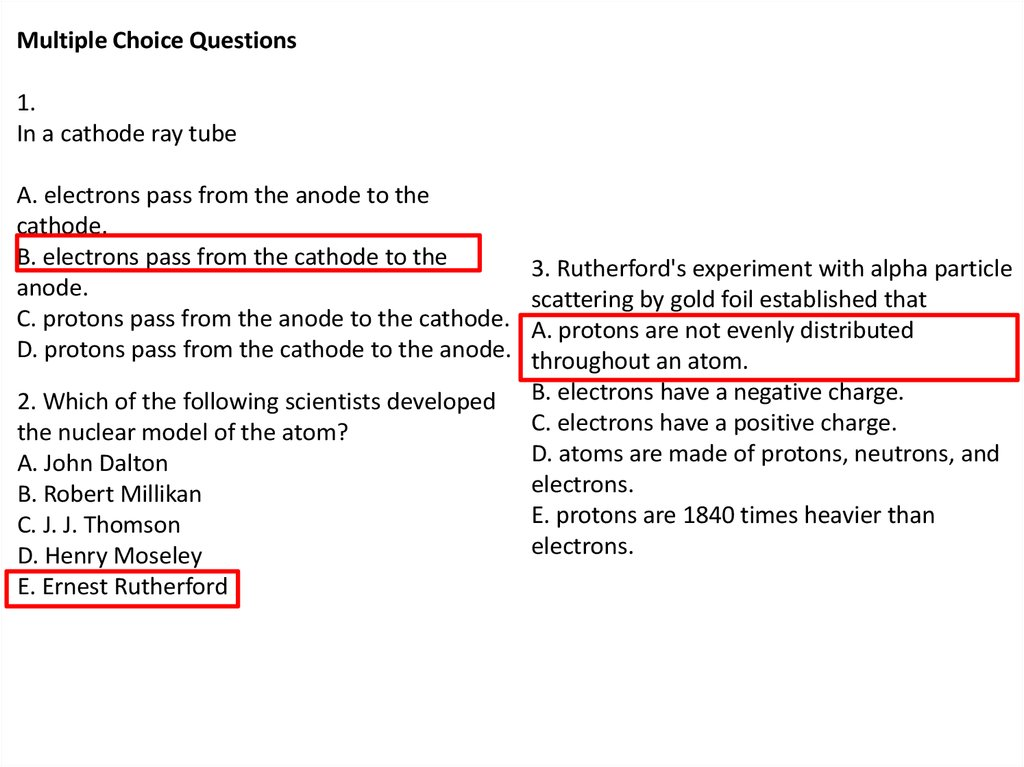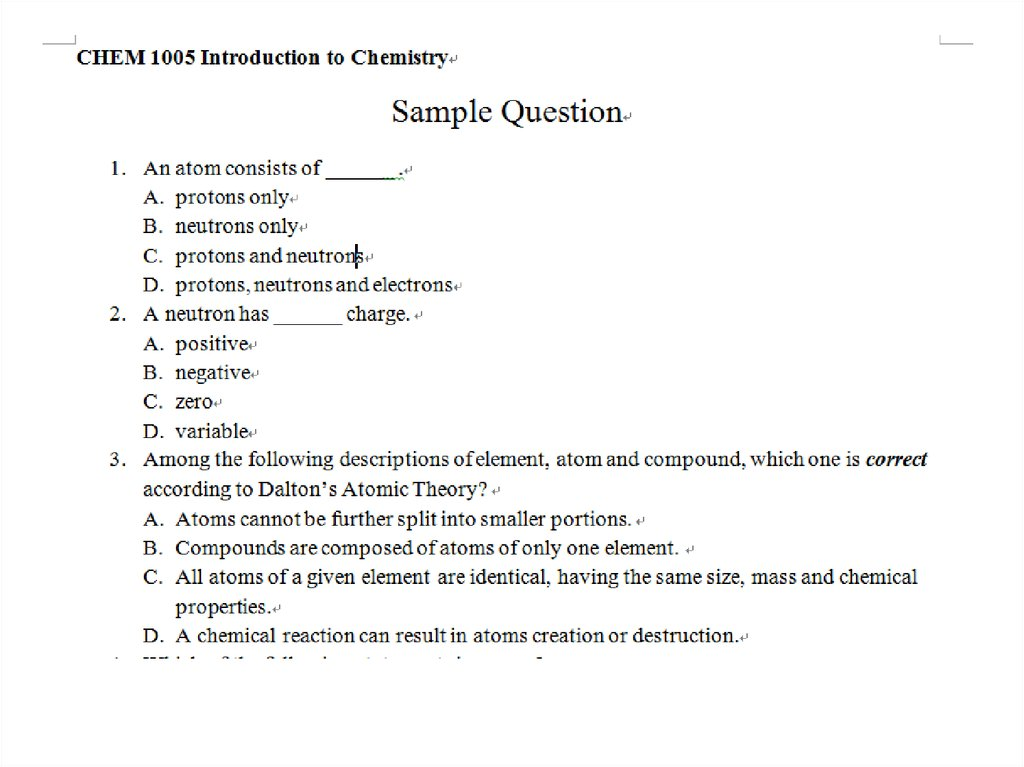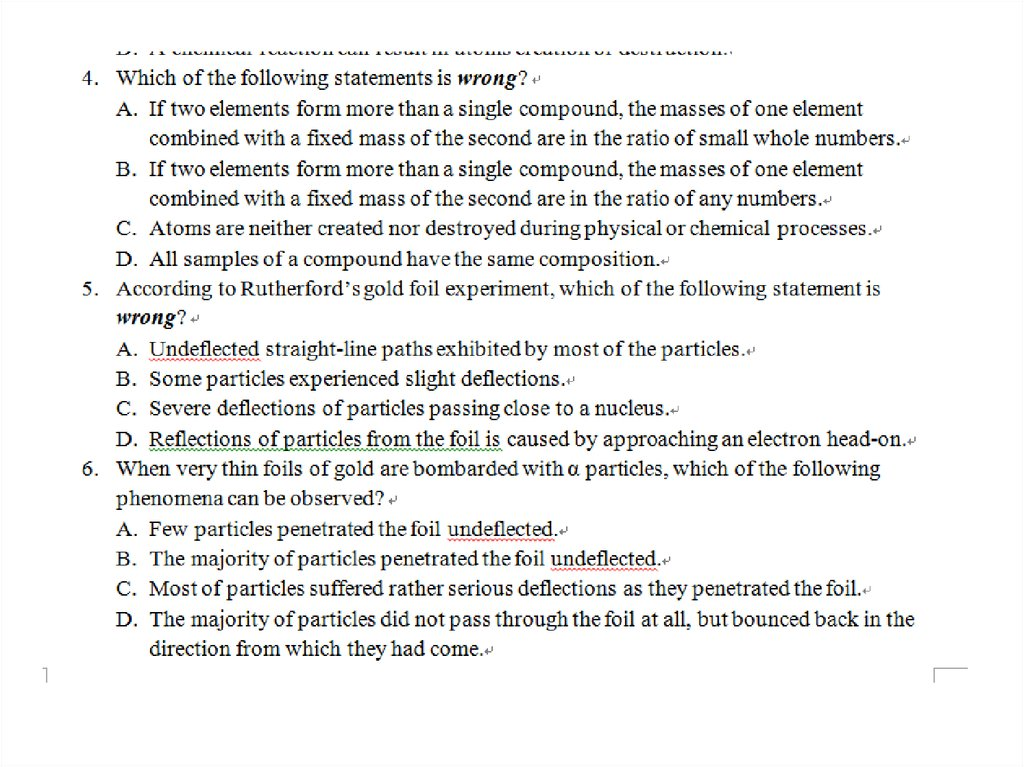# Multiple Choice Questions

## 1.

Multiple Choice Questions
1.
In a cathode ray tube
A. electrons pass from the anode to the
cathode.
B. electrons pass from the cathode to the
anode.
C. protons pass from the anode to the cathode.
D. protons pass from the cathode to the anode.
2. Which of the following scientists developed
the nuclear model of the atom?
A. John Dalton
B. Robert Millikan
C. J. J. Thomson
D. Henry Moseley
E. Ernest Rutherford
3. Rutherford's experiment with alpha particle
scattering by gold foil established that
A. protons are not evenly distributed
throughout an atom.
B. electrons have a negative charge.
C. electrons have a positive charge.
D. atoms are made of protons, neutrons, and
electrons.
E. protons are 1840 times heavier than
electrons.

## 8.

• Solution:
• The atomic number is 29, so there are 29 protons. The
mass number is 63, so the number of neutrons is 6329=34. The number of electrons is the same as the
number of protons, that is 29.

## 11.

Which of the following are isotopes?
A. 14C and 13C
B. 14C and 14N
C. 14N and 14N3D. 12C and 12CO
E. 14N and 14N2

## 12.

Complete the following chart, in order from left to right
A. 14, 7, 7, 7
B. 14, 7, 14, 7
C. 7, 7, 7, 7
D. 7, 14, 7, 7
A. 40Zr, 21
B. 19K, 40
C. 21K, 19
D. 40K, 21
E. 38Sr, 19
A. 97Zr, 97
B. 40Zr, 57
C. 57La, 40
D. 97Bk, 80
E. 80Hg, 97

## 15.

2) 70%
Solution
62.9X + 64.9 (1-X)= 63.5
-2.0 X = -1.4
X = 70%

## 21.

In the following diagram of a wave
A. (a) is amplitude and (b) is wavelength
B. (a) is frequency and (b) is amplitude
C. (a) is wavelength and (b) is frequency
D. (a) is amplitude and (b) is frequency
E. (a) is wavelength and (b) is amplitude

## 23.

Using the figure below, categorize electromagnetic radiation with a wavelength of 1.0 x 10 -3 m.
A. Gamma rays
B. X rays
C. Ultraviolet
D. Infrared
E. Microwave

## 24.

• Solution:
• Replacing u with c (the speed of light) gives
c=lxn
l= c/ n
= 3.00 x 108 m/s / 3.64 x 107 Hz
= 3.00 x 108 m/s / 3.64 x 107 /s
= 8.24 m

## 25.

Which of the following wavelengths of
energy?
A. 450. nm
B. 225 nm
C. 3.50 x 10-9 m
D. 8.40 x 10-7 m
E. 2.50 x 10-5 m

## 29.

• Solution:
E = h x c/ l
l = h x c/ E
= 6.63 x 10-34 (J•s) x 3.00 x 108 (m/s) / 5.87 x 10-20 J
= 3.39 x 10-6 m
= 3.39 x 103 nm

• Solution: